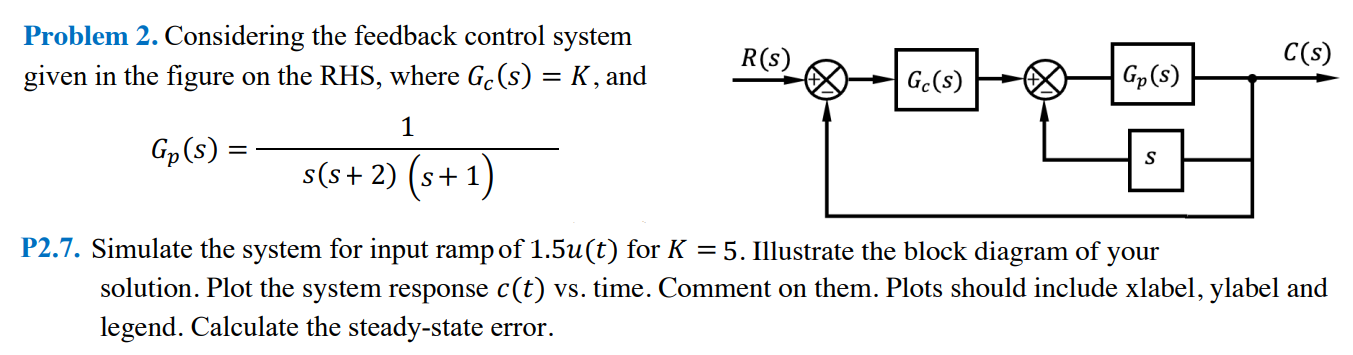# (Solved): Please add MATLAB codes, illustrate the block diagram with plotting the sy ...???????Please add MATLAB codes, illustrate the block diagram with plotting the sytem response including xlabel ylabel and legend. And please calculate steady state error. Thank you.

Problem 2. Considering the feedback control system given in the figure on the RHS, where $$G_{c}(s)=K$$, and $G_{p}(s)=\frac{1}{s(s+2)(s+1)}$ P2.7. Simulate the system for input ramp of $$1.5 u(t)$$ for $$K=5$$. Illustrate the block diagram of your solution. Plot the system response $$c(t)$$ vs. time. Comment on them. Plots should include xlabel, ylabel and legend. Calculate the steady-state error.

We have an Answer from Expert